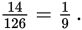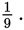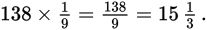# SAT Math Multiple Choice Question 413: Answer and Explanation

### Test Information

Question: 413

8. A student is doing a scale drawing of a woolly mammoth on a piece of poster board for her presentation on the last ice age. She was surprised to find that the woolly mammoth, reaching a height of only about 10 feet, 6 inches, was actually smaller than today's African elephant. Even more surprising is the fact that the woolly mammoth's tusks averaged 11.5 feet in length. If the student draws the mammoth 14 inches tall on her poster, approximately how many inches long should she make the tusks?

• A. 12.78
• B. 15.0
• C. 15.33
• D. 16.1

Getting to the Answer: There are 12 inches in one foot, so the height of the woolly mammoth was 10 × 12 = 120 + 6 = 126 inches. The tusk length was 11.5 feet, or 11.5 × 12 = 138 inches. The student plans to draw the mammoth 14 inches tall, so find the scale factor of the two heights by writing them as a fraction:This means the scale factor isMultiply this by the length of the real mammoth's tusks to find the scaled length:This means the student should make the tusks 15.33 inches long.